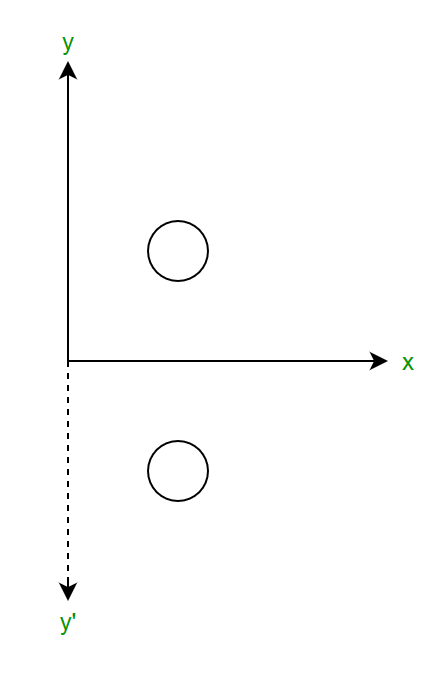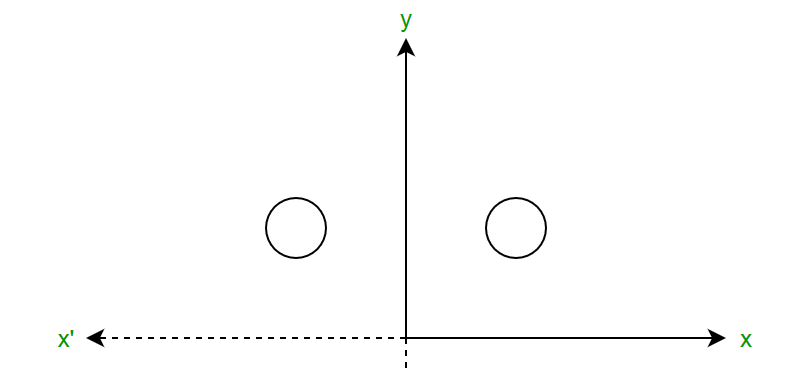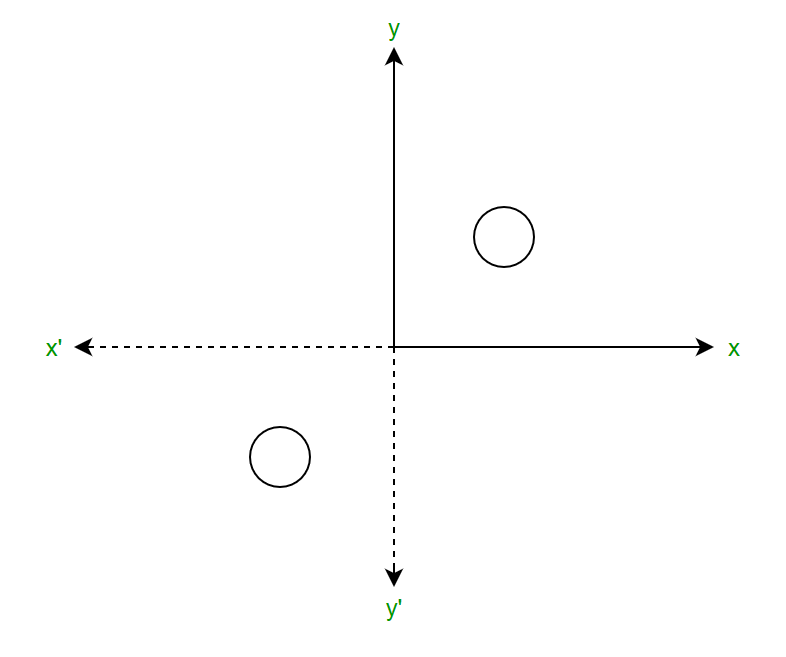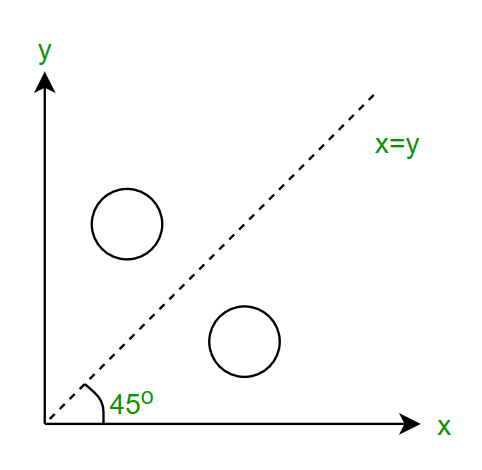# Reflection In 2D Graphics

Reflection deals with obtaining a mirror image of the 2D object.

If P(x, y) is the point on x-y plane then P’(x’, y’) is the reflection about x-axis given as x’=x ; y’=-yMatrix Form:If P(x, y) is the point on x-y plane then P’(x’, y’) is the reflection about y-axis given as x’=-x ; y’=yAlong origin :
If P(x, y) is the point on x-y plane then P’(x’, y’) is the reflection about origin given as x’=-x ; y’=-yAbout x=y line : To do this move x=y line to any of the axis. In the given diagram the angle of rotation is 45o as the points are plotted as (0, 0), (1, 1), (2, 2), and so on.Imposing the line clockwise (-45o) imposing it on the x-axis we have,We know,andNow perform reflection along x-axis,Now rotate the line back 45o in an anticlockwise direction,Now if P(x, y) is the point on x-y plane then P’(x’, y’) is the reflection about x=y line given as x’=y ; y’=x
Matrix Form:My Personal Notes arrow_drop_upCheck out this Author's contributed articles.

If you like GeeksforGeeks and would like to contribute, you can also write an article using contribute.geeksforgeeks.org or mail your article to contribute@geeksforgeeks.org. See your article appearing on the GeeksforGeeks main page and help other Geeks.

Please Improve this article if you find anything incorrect by clicking on the "Improve Article" button below.

Article Tags :
Practice Tags :

Be the First to upvote.

Please write to us at contribute@geeksforgeeks.org to report any issue with the above content.